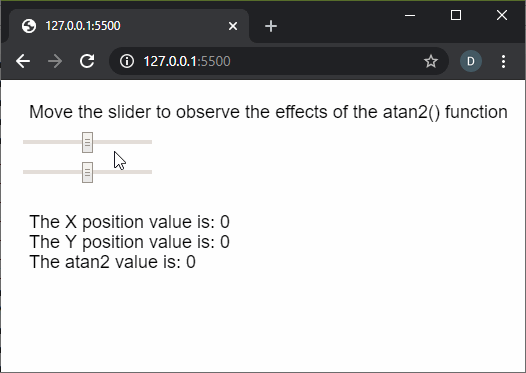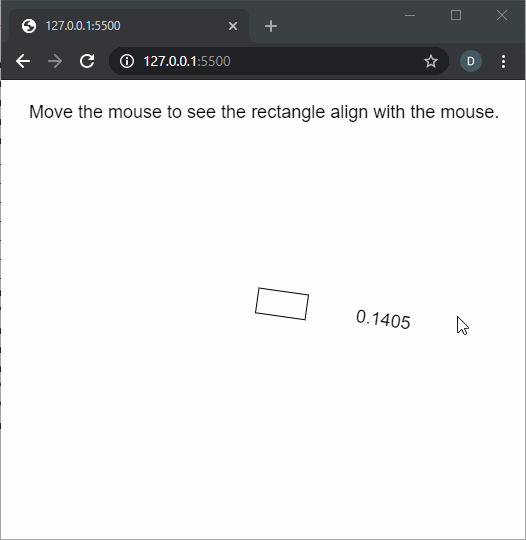# p5.js | atan2() Function

• Last Updated : 21 Apr, 2020

The atan2() function in p5.js is used to calculate the angle from a specified point of origin measured from the positive x-axis. The values are returned in the range of PI to -PI as floating points. It is commonly used for orienting geometry to the position of the cursor.

Syntax:

Hey geek! The constant emerging technologies in the world of web development always keeps the excitement for this subject through the roof. But before you tackle the big projects, we suggest you start by learning the basics. Kickstart your web development journey by learning JS concepts with our JavaScript Course. Now at it's lowest price ever!

`atan2(y, x)`

Parameters: This function accepts two parameters as mentioned above and described below.

• y: It is a number that specifies the y-coordinate of the point.
• x: It is a number that specifies the x-coordinate of the point.

Return Value: It returns a number that denotes the arc tangent of the given point.

Below examples illustrate the atan2() function in p5.js:

Example 1:

 `function` `setup() {``  ``createCanvas(500, 200);``  ``textSize(18);`` ` `  ``text(``"Move the slider to observe the effects"``         ``+ ``" of the atan2() function"``, 20, 30);`` ` `  ``sliderXPos = createSlider(-200, 200, 0, 1);``  ``sliderXPos.position(20, 50);`` ` `  ``sliderYPos = createSlider(-200, 200, 0, 1);``  ``sliderYPos.position(20, 80);``}`` ` `function` `draw() {``  ``clear();``  ``text(``"Move the slider to observe the effects"``         ``+ ``" of the atan2() function"``, 20, 30);`` ` `  ``sliderXVal = sliderXPos.value();``  ``sliderYVal = sliderYPos.value();`` ` `  ``atan2Val = atan2(sliderXVal, sliderYVal);`` ` `  ``text(``"The X position value is: "` `+ sliderXVal, 20, 140);``  ``text(``"The Y position value is: "` `+ sliderYVal, 20, 160);``  ``text(``"The atan2 value is: "` `+ atan2Val, 20, 180);``}`

Output:Example 2:

 `function` `setup() {``  ``createCanvas(500, 400);``  ``textSize(18);``  ` `  ``text(``"Move the mouse to see the rectangle"``        ``+ ``" align to it."``, 20, 30);``}``  ` `function` `draw() {``  ``clear();``  ``text(``"Move the mouse to see the rectangle"``        ``+ ``" align with the mouse."``, 20, 30);``  ` `  ``// Move the rectangle to the``  ``// middle of the screen``  ``translate(width / 2, height / 2);``  ` `  ``// Use the atan2() function to find``  ``// the value according to the mouse``  ``// coordinates``  ``let adjustedValue = atan2(mouseY - height / 2,``                            ``mouseX - width / 2);``  ``rotate(adjustedValue);``  ` `  ``// Draw a rectangle``  ``rect(0, 0, 50, 25);``  ` `  ``text(adjustedValue.toFixed(4), 100, 20);``}`

Output:Online editor: https://editor.p5js.org/

Reference: https://p5js.org/reference/#/p5/atan2

My Personal Notes arrow_drop_up